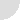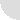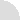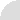LOGOLOG
a weblog of wordplay by Eric Harshbarger

## Slices of PI, Part 1

In this day and age a lot of logology centers around computer analysis of long wordlists, word configurations, and the like. Often the challenge really is in writing a program which will conduct an analysis in a reasonable time. "Reasonable" may still mean months of CPU time, but years (or BILLIONS of years) is probably in the "unreasonable" category. Figuring out the best way to scan for word squares, for example, is a non-trivial task.

Using a computer is almost always faster than hunting by hand, but a programmer still wants to make it as efficient as possible.

Scanning large lists of words is exactly what computers excel at, but sitting in front of one, staring at the screen, waiting for a result to pop out is still very boring.

If there's one group of folks who implement monotonous scanning programs at least as often as recreational linguists, it's recreational mathematicians. The guys who are scanning for the largest primes numbers are a, ahem, prime example of this (forgive me, guys, I don't mean to trivialize the search by labelling it "recrational" or simply "scanning" -- I'm a mathematiciam by education, and hold such projects in as high regard as logology).

Another example of true number crunching is the generation of umpteen digits of PI (the ratio of a circle's circimference to its diameter, roughly 3.1415926...).

I thought it would be interesting to use PI and its decimal expansion as a way to join my interests in recreational wordplay and numberplay. So here goes...

When I refer to "the digits of PI" and such, I will actually just be talking about all of the numbers after the decimal point (1415926...). This is one of many arbitrary parameters I'm about to define for the task below. I had to make a decision whether to include the beginning "3." or not, and for no reason at all, really, I have chosen not to.

Now, let's define a length, L, and a value called a slice size, S. L will refer to a particular word length, while S is the size of a string of digits extracted from the decimal expansion of PI.

We will be interested in the first L slices of PI, each of length S.

So, if I choose my word length to be L=4, and slice size to be S=7, then the digits of PI that will be employed will be the fist 28 digits of PI:

```1415926 5358979 3238462 6433832
```
Split the 28 digits into four groups of 7 (in general, L groups of size S). In this case we are then looking at four 7-digit numbers. Now divide each of those four numbers by 26 and throw away everything but the remainder (we are performing modular arithmetic using a modulus of 26).

Your remainder will be a number inclusively between 0 and 25 (zero if 26 divides the seven digit number evenly).

Assign the letters of the alphabet index values so that A=0, B=1, C=2, ..., Z=25 (this is a bit different than the way often used in which A=1 and Z=26).

For each of the fout 7-digit numbers, then, we have assigned a letter of the alphabet. In our example when get:

```1415926 % 26 = 18 = S ("%" indicates modular arithmetic)
5358979 % 26 = 15 = P
3238462 % 26 = 6  = G
6433832 % 26 = 2  = C
```

Our "word" of length 4 is: "SPGC".

Of course, this is not a real word, not one that you'd find in any respectable wordlist. But, you get the idea of what could be done. This example used particular values for L and S. But there are many others... infinitely many others.

For what values of L and S do we actually generate words?

That is the challenge I put forth to you, the reader.

I'll give you a valid example. When L=2 and S=1, then we have the following answer:

```1 % 26 = 1 = B
4 % 26 = 4 = E
```
We end up with the 2-length word "BE". Woohoo! A real word. What about the other words of length 2? Longer?

Obviously, to tackle this challenge, you'll need to be able to program computers to do most of the work. You can do a bit by hand, but soon you'll find yourself wanting to divide 1000-digits numbers by hand (and much longer!)... you should NOT try this if you want to keep your sanity intact.

Computers can do this, however, if coaxed with the proper lines of code.

Once I established the rules above, I wrote such an analysis application, and started crunching numbers with slice sizes up to 10,000 digits in length. These were the preliminary results I got (using the tournament Scrabble wordlist based on OSPD4):

• All 101 words of length two (L=2) were generated with slice sizes under ten thousand digits. The first was BE at S=1, the last was MU all the way up at S=4852 (that means the program was dividing a four thousand, eight hundred and fifty-two digit number by 26).
• EYE was the earliest 3-length word found (at S=11). A total of 433 (out of 1015 words of this length) made an appearance below the 10,000 threshold, with TAV sneaking in at S=9979.
• Only 106 four letter words appeared within the 10,000 threshold (WEIR at S=268, HOWL at S=9986).
• Five letter words were even rarer. Only 6 came through below 10,000 (CANAL was the earliest at S=600).
At this point I thought I'd do a couple of things:

First, I decided to establish a shorthand notation for all of this. In a mathematical sense, I defined a function pi() which assigns a value to a word. The value is equal to the smallest slice size which generates that word as defined by the rules above (I say smallest, because larger slices may, in fact, generate the same word again). So, shorthand notation for some of the examples above would be:

```pi(BE) = 1
pi(HOWL) = 9986
pi(CANAL) = 600
```

Next, I decided I should have someone verify my results thus far. I feel pretty confident writing programs like this (which are pretty straightforward), but when you start asking a computer to divide 10000-digit numbers, it never hurts to see if someone else's computer gets the same results.

I got in touch with another word and numberplay guy I'd heard about, Mike Keith. I asked if he'd be interested in verifying my results.

Boy, was he interested. A couple of days later he responded not only with verification (my answers were correct), but he had downloaded the first 5 million digits of PI and ran calculations through word lengths of seven! His maximum slice sizes varied, of course, depending on the word length. With five million digits to play with, four letter words would let him achieve a maximum slice size of S=1,250,000 (5,000,000 / 4). The larger the word length the lower the maximum slice size.

Here is a summary of the results he generated (some verifying what I had done):

 L Max (S) # words within threshold Earliest word Latest word 2 2,500,000 101* pi(BE) = 1 pi(MU) = 4852 3 1,666,666 1015* pi(EYE) = 11 pi(MOB) = 114,950 4 1,250,000 3797 pi(WEIR) = 268 pi(BOGY) = 1,249,799 5 1,000,000 704 pi(CANAL) = 600 pi(DELTS) = 997,391 6 833,333 33 pi(WHARVE) = 46441 pi(GLIFFS) = 819,309 7 714,285 1 pi(ABLEIST) = 89,482 pi(ABLEIST) = 89,482 * indicates that all the words of length L were found using a slice size of Max(S) or less.

Again, discoveries drop off quickly. Only one seven-letter word was found using the first five million digits of PI (and it came relatively quickly: at s=89482).

This might seem discouraging, but keep in mind, we're not going to run out of digits of PI. You can find statistics about the first 1.2 trillion digits of PI on the web, and that's just with a quick search. Of course, the above wordplay task will quickly crunch through all of those digits if you have access to a powerful enough machine.

But PI goes on and on...

We will never know all of its digits, and we can't even predict what the next digit will be. So, while a trillion digits might get used up quickly... one needs to keep in mind that in the mathematical scheme of things, in the infinite scheme of things, a trillion digits is nothing... not even a drop in the bucket. Neither is a trillion, trillion, trillion, digits... any finite number, regardless of its size, can't hold a match to the infinity of PI. It soon becomes a speck.

So while only one seven letter words (and a strange on at that, ABLEIST) has been found so far in this wordplay challenge, others are out there to be found. In fact, all of them are out there... somewhere hidden in PI. PI's digits are essentially random, and, again, infinite. If we search long enough, with large enough slice sizes, we will find all of the seven letters words.

We'd also find all of the eight letter words, too. And 9s, 10s, et cetera.

Infinity can be hard to grasp at times like this.

If we could search with extraordinarily large slice sizes we would eventually find the phrase, "MARY HAD A LITTLE LAMB" (ignoring spaces). We'd find the whole poem embedded in PI, too.

We would even find the complete works of Shakespeare.

Have I made my point [grin]?

But right now, we have only found up to one seven letter word.

There's still work to be done!

-- Eric

P.S.: in the next entry of LOGOLOG I'll talk a bit more about the mathematics of finding valid words, and the arbitrariness of the rules I established above.

[11 January 2006]Archive```Your Name:

Your comment (HTML tags will be removed):

Day of week today is:

```